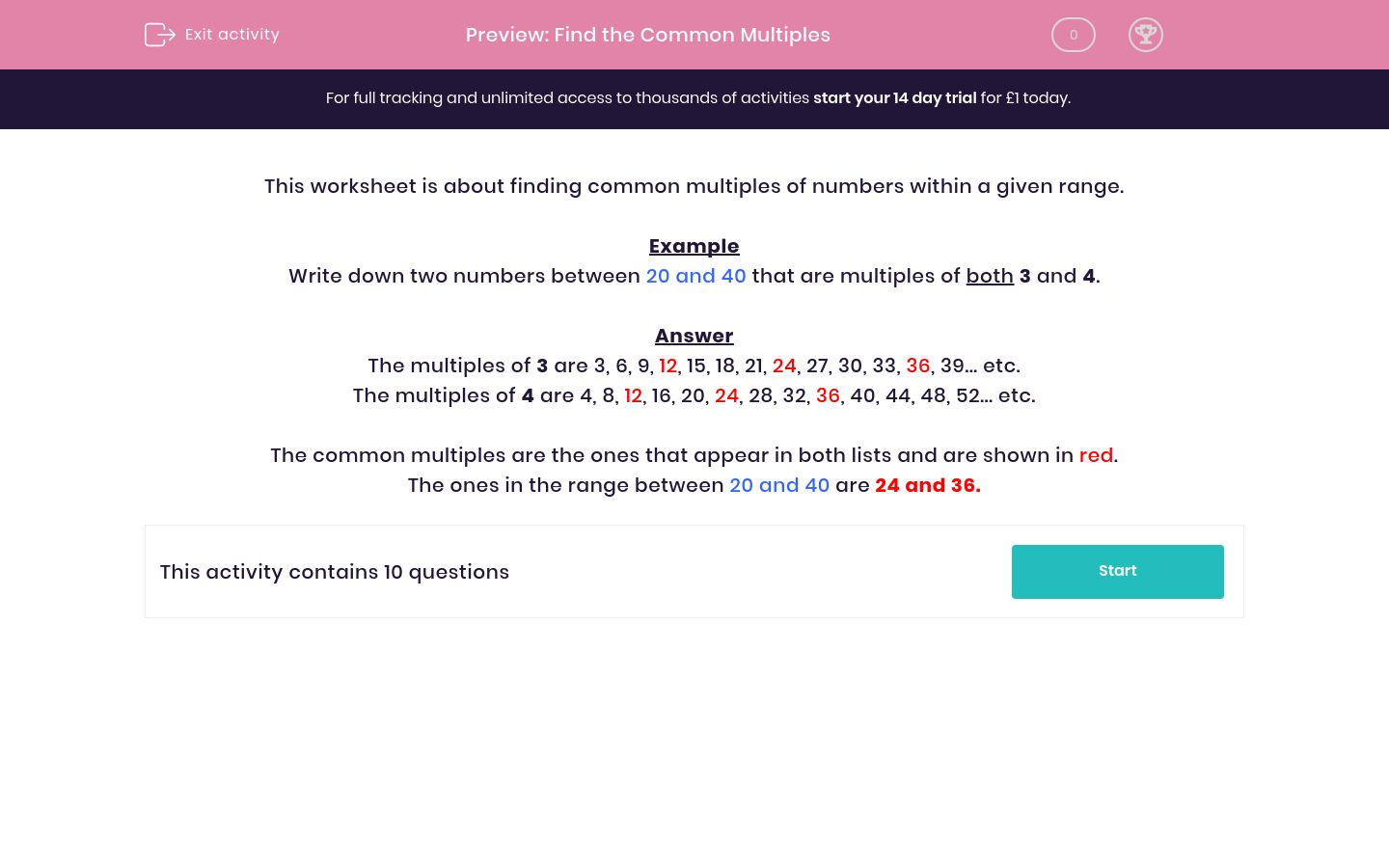# Find the Common Multiples

In this worksheet, students find two common multiples of numbers within a given range.Key stage:  KS 2

Curriculum topic:   Maths and Numerical Reasoning

Curriculum subtopic:   Factors and Multiples

Difficulty level:### QUESTION 1 of 10

This worksheet is about finding common multiples of numbers within a given range.

Example

Write down two numbers between 20 and 40 that are multiples of both 3 and 4.

The multiples of 3 are 3, 6, 9, 12, 15, 18, 21, 24, 27, 30, 33, 36, 39... etc.

The multiples of 4 are 4, 8, 12, 16, 20, 24, 28, 32, 36, 40, 44, 48, 52... etc.

The common multiples are the ones that appear in both lists and are shown in red.

The ones in the range between 20 and 40 are 24 and 36.

Write down two numbers less than 50 that are multiples of both 8 and 6.

 Number Lowest common multiple Highest common multiple

Write down two numbers less than 90 that are multiples of both 11 and 3.

 Number Lowest common multiple Highest common multiple

Write down two numbers less than 10 that are multiples of both 2 and 4.

 Number Lowest common multiple Highest common multiple

Write down two numbers less than 30 that are multiples of both 10 and 5.

 Number Lowest common multiple Highest common multiple

Write down two numbers between 20 and 90 that are multiples of both 3 and 10.

 Number Lowest common multiple Highest common multiple

Write down two numbers between 20 and 90 that are multiples of both 5 and 6.

 Number Lowest common multiple Highest common multiple

Write down two numbers between 10 and 20 that are multiples of both 6 and 2.

 Number Lowest common multiple Highest common multiple

Write down two numbers less than 40 that are multiples of both 3 and 5.

 Number Lowest common multiple Highest common multiple

Write down two numbers between 20 and 50 that are multiples of both 3 and 7.

 Number Lowest common multiple Highest common multiple

Write down two numbers between 20 and 90 that are multiples of both 6 and 5.

 Number Lowest common multiple Highest common multiple
• Question 1

Write down two numbers less than 50 that are multiples of both 8 and 6.

 Number Lowest common multiple Highest common multiple
EDDIE SAYS
The common multiples are 24 and 48.
• Question 2

Write down two numbers less than 90 that are multiples of both 11 and 3.

 Number Lowest common multiple Highest common multiple
EDDIE SAYS
The common multiples are 33 and 66.
• Question 3

Write down two numbers less than 10 that are multiples of both 2 and 4.

 Number Lowest common multiple Highest common multiple
EDDIE SAYS
The common multiples are 4 and 8.
• Question 4

Write down two numbers less than 30 that are multiples of both 10 and 5.

 Number Lowest common multiple Highest common multiple
EDDIE SAYS
The common multiples are 10 and 20.
• Question 5

Write down two numbers between 20 and 90 that are multiples of both 3 and 10.

 Number Lowest common multiple Highest common multiple
EDDIE SAYS
The common multiples are 30 and 60.
• Question 6

Write down two numbers between 20 and 90 that are multiples of both 5 and 6.

 Number Lowest common multiple Highest common multiple
EDDIE SAYS
The common multiples are 30 and 60.
• Question 7

Write down two numbers between 10 and 20 that are multiples of both 6 and 2.

 Number Lowest common multiple Highest common multiple
EDDIE SAYS
The common multiples are 12 and 18.
• Question 8

Write down two numbers less than 40 that are multiples of both 3 and 5.

 Number Lowest common multiple Highest common multiple
EDDIE SAYS
The common multiples are 15 and 30.
• Question 9

Write down two numbers between 20 and 50 that are multiples of both 3 and 7.

 Number Lowest common multiple Highest common multiple
EDDIE SAYS
The common multiples are 21 and 42.
• Question 10

Write down two numbers between 20 and 90 that are multiples of both 6 and 5.

 Number Lowest common multiple Highest common multiple
EDDIE SAYS
The common multiples are 30 an 60.
---- OR ----

Sign up for a £1 trial so you can track and measure your child's progress on this activity.

### What is EdPlace?

We're your National Curriculum aligned online education content provider helping each child succeed in English, maths and science from year 1 to GCSE. With an EdPlace account you’ll be able to track and measure progress, helping each child achieve their best. We build confidence and attainment by personalising each child’s learning at a level that suits them.

Get started